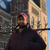# QlikView App Dev

Discussion Board for collaboration related to QlikView App Development.

Announcements
Customer & Partners, DEC. 9, 11 AM ET: Qlik Product & Strategy Roadmap Session: Data Analytics REGISTER NOW
cancel
Showing results for
Did you mean:Contributor III

## Aggregation issue in Straight Table/Pivot Table

Hi,

I have an particular requirement where i am trying to aggregate the data based on an One dimension. There are two dimensions in Total for the same graph. The Sum of the Values is getting calculated properly for this Year and Last Year. But i need to calculate the sum based on division as well and divide the individual numbers by this cumulative sum to get the percentage.

i.e for instance Last Year Under Division A say i am getting 20 for Container A and 40 for Container B and 40 for Container C then i need to get 20 % for A's Share and 40% for B's Share and 40 % for C's Share. But the Problem is that the Aggregation is not happening properly under Division Alone. I need to specify the ContainerType as well which will be common for Division B and Division C as well

Please Note : The First Dimension is DivisionNaming(based on which Aggregation Should Happen) and the second dimension is ContSize. If i remove ContSize it is correct. But need that dimension as well

Attached an Sample Excel.

The Formula that i am using is below

((Sum({<FinYear={'\$(=MAX(FinYear)-1)'},originalCostingDate= {">=\$(=MonthStart(today()-365))<=\$(=today()-365)"},ContType=,ContSize=>} LineTEU))/

Sum(Aggr(Sum({<FinYear={'\$(=MAX(FinYear)-1)'},originalCostingDate= {">=\$(=MonthStart(today()-365))<=\$(=today()-365)"},ContType=,ContSize=>}TOTAL LineTEU),DivisionNaming1))

)

Thanks in Advance for the help.

Thanks and Regards,

Vigneshwaran.M.K

4 RepliesChampion

Hi,

I can't find the Measure_field used on your expression and the dimension name used in the aggr() function above in the file you have attached:

Measure field :LineTEU

Dimension: DivisionNaming1Contributor III
Author

Hi Youssef,

Thanks For your Email. Yes both are correct . Also i am using Second Dimensions as ContSize.

The Problem is that there are three divisions and all three divisions will have all the three Cont Size.

So this % Share column should be the Sum of the Value for that Row / Sum of the value for that whole Division

So i am trying to so the same by giving the numerator as Sum and Denominator as Aggr(Sum(),Division).

The Problem is that Since we have another Value in Dimension it is not happening Properly. But i need the Second Dimension as wellChampion

I think you misunderstood me

in your attached file, where is the field: LineTEU ??

you're making the sum on that fieldContributor II

Hi vigneshwaran,

Try the below expressions

((Sum({<FinYear={'\$(=MAX(FinYear)-1)'},originalCostingDate= {">=\$(=MonthStart(today()-365))<=\$(=today()-365)"},ContType=,ContSize=>} LineTEU))/

((Sum({<FinYear={'\$(=MAX(FinYear)-1)'},originalCostingDate= {">=\$(=MonthStart(today()-365))<=\$(=today()-365)"},ContType=,ContSize=>} Total<DivisionNaming1>LineTEU))

)

Basically for numerator you want to total as per the Division naming field.In total you could pass the fields from the chart which should only be considered for calculation.

Hope this helps.Community Browser